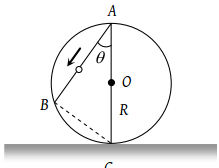A frictionless wire AB is fixed on a sphere of radius R. A very small spherical ball slips on this wire. The time taken by this ball to slip from A to B is(1) $\frac{2\sqrt{gR}}{g\mathrm{cos}\theta }$

(2) $2\sqrt{gR}.\frac{\mathrm{cos}\theta }{g}$

(3) $2\sqrt{\frac{R}{g}}$

(4) $\frac{gR}{\sqrt{g\mathrm{cos}\theta }}$

Concept Videos :-

#26-Equations-for-Uniform-Acceleration
#27-Equations for Uniform Acceleration 28Calculus Method29
#28-Sign Convention
#29-Solved Examples 16
#31-Solved-Examples-18
#32-Solved Examples 19
#33-Solved Examples 20
#34-Solved-Examples-21
#35 Solved Examples 22
#30-Solved Examples 17

Concept Questions :-

Uniformly accelerated motion

(3) Acceleration of body along AB is $g\mathrm{cos}\theta$

Distance travelled in time t sec = $AB=\frac{1}{2}\left(g\mathrm{cos}\theta \right){t}^{2}$

From $\Delta ABC,\text{\hspace{0.17em}}AB=2R\mathrm{cos}\theta ;\text{\hspace{0.17em}}2R\mathrm{cos}\theta =\frac{1}{2}g\mathrm{cos}\theta {t}^{2}$

${t}^{2}=\frac{4R}{g}$ or $t=2\sqrt{\frac{R}{g}}$

Difficulty Level:

• 12%
• 20%
• 64%
• 6%